## Problem in ploting data

Hello community,

I want plot data using proc timeseries

```proc timeseries data=data plots= series;

id date interval=YEAR;
var   Debt;
run;```

but i didn't get the diagram.

1 ACCEPTED SOLUTION

Accepted Solutions

## Re: Problem in ploting data

@dw_sas was right in his suggestion of how to fix your problem.

But you still have a few issues in your code.

• Do not use the same data set name and the SET name. It makes it really hard to debug your code, is confusing and bad practice.
• ``````data data;
set data; ``````
• You have a year variable which is numeric and represents a year, as 1994, 1995. It is not a SAS date.
• For some reason you think you need to loop - SAS does this automatically so it's a single line of code to create your year variable.
• ``````%let start=1983;
%let end=2017;

data data;
set data;
format date year4. ;
date = "01jan&start."d;
do until (date > "01jan&end."d);
output data;
date = intnx('year',date,1);
end;
run; ``````
• When I imported the data there were some empty rows and columns, I deleted them.

I changed the variable name from date to myDate to make the code more clear.

See below:18 REPLIES 18

## Re: Problem in ploting data

If the suggestions you got in https://communities.sas.com/t5/SAS-Forecasting-and-Econometrics/Error-in-date-variable/m-p/492797 did not provide the necessary solution, please provide example data in usable form (datastep with datalines), and describe what you want to get out of it (and/or show an example for that). It might be that proc timeseries is not what you need at all.

## Re: Problem in ploting data

When i want plot data i get error that the variable date is not defined.

After resolving the first problem thanks to you and i want plot data with that code i got only this table.

This is the data

## Re: Problem in ploting data

Please revise your question.  Provide test data in the form of a datastep:

https://communities.sas.com/t5/SAS-Communities-Library/How-to-create-a-data-step-version-of-your-dat...

only need a few rows which accurately describe the problem.

Next post the exact code you are using - use the code window, it is the {i} above post area, to retain formatting.

Next post the log of the run part in question, again use the code window.

A quick glance at the Excel file you posted, shows that there is no variable called date, therefore you get the error because you have tried to use a variable which is not in the data in:

`   id date interval=YEAR;      ^here`

I would also advice you stop using SAS keywords as names, data=data for instance is just bad coding, and calling a date variable date, will just get very confusing.

## Re: Problem in ploting data

The date variable in excel is named Year. When i want plot the data using this variable i got the message:

"ERROR: Duplicate time interval found at observation number 2 in the data set WORK.DATA, according to
the INTERVAL=YEAR option and the ID variable values. The current ID is Year=1984 and the
previous is Year=1983, which are within the same YEAR interval.
Check that INTERVAL=YEAR is correct for this data set, and that the ID variable Year contains
SAS date or datetime values that correctly identify the observations"

So i created new variable date named date using code in ancient post and i used the code to plot data i got

"ERROR: The data set WORK.DATA is not sorted by the ID variable. At observation number 36, date=1983,
but date=2017 for the previous observation."

I sorted the variable by date but when i used code to plot it i got

"ERROR: Observation with duplicate ID value found. The value of the ID variable, date=1983, at
observation number 2 in data set WORK.DATA is the same as the previous observation."

## Re: Problem in ploting data

@Lok07 wrote:

The date variable in excel is named Year. When i want plot the data using this variable i got the message:

"ERROR: Duplicate time interval found at observation number 2 in the data set WORK.DATA, according to
the INTERVAL=YEAR option and the ID variable values. The current ID is Year=1984 and the
previous is Year=1983, which are within the same YEAR interval.
Check that INTERVAL=YEAR is correct for this data set, and that the ID variable Year contains
SAS date or datetime values that correctly identify the observations"

So i created new variable date named date using code in ancient post and i used the code to plot data i got

"ERROR: The data set WORK.DATA is not sorted by the ID variable. At observation number 36, date=1983,
but date=2017 for the previous observation."

I sorted the variable by date but when i used code to plot it i got

"ERROR: Observation with duplicate ID value found. The value of the ID variable, date=1983, at
observation number 2 in data set WORK.DATA is the same as the previous observation."

## Re: Problem in ploting data

this is the code

```proc import out=data
datafile="C:\Users\hp\Desktop\debt.xlsx"
dbms=excel replace; getnames=yes;
run;
proc timeseries data=data plots= series;
id Year interval=YEAR;
var   Debt;
run;
%let start=1983;
%let end=2017;

data data;
set data;
format date year4. ;
date = "01jan&start."d;
do until (date > "01jan&end."d);
output data;
date = intnx('year',date,1);
end;
run;
proc sort data=data;
by date;
run;
proc timeseries data=data plots= series;
id date interval=YEAR;
var   Debt;
run;```

## Re: Problem in ploting data

Hello,

In order to better understand the source of the problem you are encountering, please provide the following information:

1) The "Alphabetic List of Variables and Attributes" table from the output of PROC CONTENTS run on your data set, DATA

2) Output of PROC PRINT on the first 10 observations of your data set, DATA

3) Details on what you are trying to plot--daily or annual data.  The Excel file contains daily data, but you are specifying INTERVAL=YEAR in the ID statement of PROC TIMESERIES.  Are you trying to accumulate the daily data to annual data prior to plotting it?  If so, then how do you want to accumulate the data (ie. annual totals, averages, etc.)?

The first two steps can be accomplished with the following code:

``````proc contents data=work.data;
run;

proc print data=work.data(obs=10);
run;``````

Once we have this information, we will be better able to address your question.

Thanks,

DW

## Re: Problem in ploting data

I want to plot annual data.

When i created a new variable date the format of this variable is YEAR4.

## Re: Problem in ploting data

Code

```proc import out=data
datafile="C:\Users\hp\Desktop\debt.xlsx"
dbms=excel replace; getnames=yes;
run;
proc timeseries data=data plots= series;
id Year interval=YEAR;
var   Debt;
run;
%let start=1983;
%let end=2017;

data data;
set data;
format date year4. ;
date = "01jan&start."d;
do until (date > "01jan&end."d);
output data;
date = intnx('year',date,1);
end;
run;
proc sort data=data;
by date;
run;
proc timeseries data=data plots= series;
id date interval=YEAR;
var   Debt;
run;```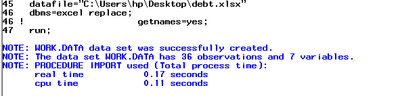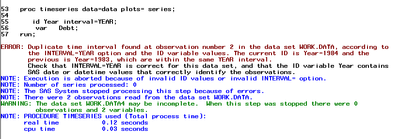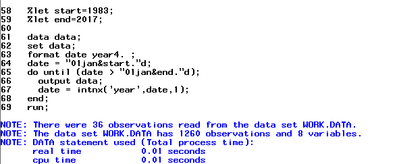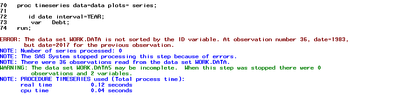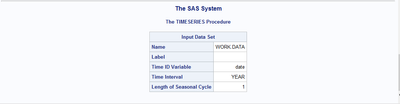## Re: Problem in ploting data

Hi,

After you import your annual data, the YEAR variable is a simple numeric year.  PROC TIMESERIES expects the ID variable to be a SAS date or datetime variable.  To create a SAS date variable from your numeric YEAR variable, please run the following code immediately after your PROC IMPORT step.  The MDY function will create a SAS date variable aligned to January 1 of each year in your YEAR variable.  Once you have this new DATE variable, you can use it in the ID statement in PROC TIMESERIES:

``````data debt_data;
set data;
date=mdy(1,1,year);
format date year4.;
run;

proc timeseries data=debt_data plots= series;
id date interval=YEAR;
var Debt;
run;``````

I hope this helps!

DW

## Re: Problem in ploting data

i got the same problem without diagram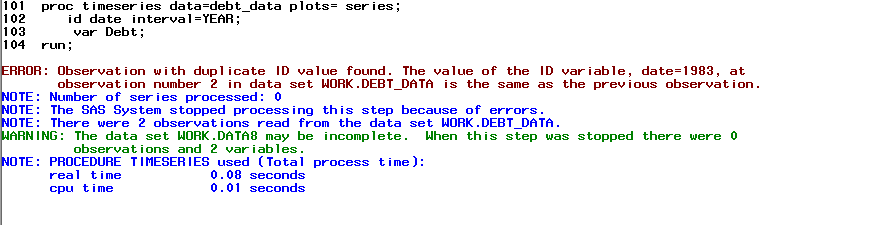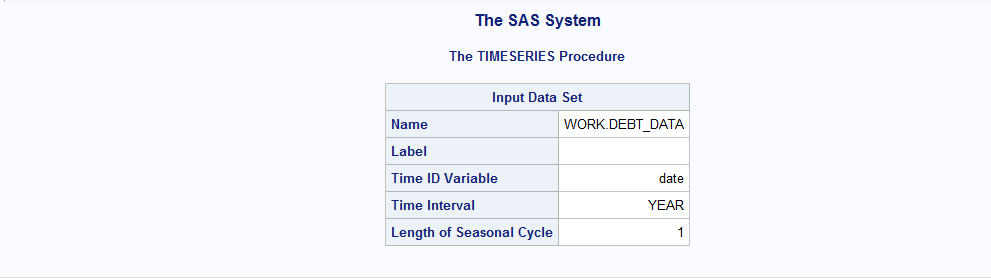Discussion stats
• 18 replies
• 1868 views
• 3 likes
• 5 in conversation